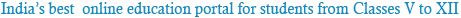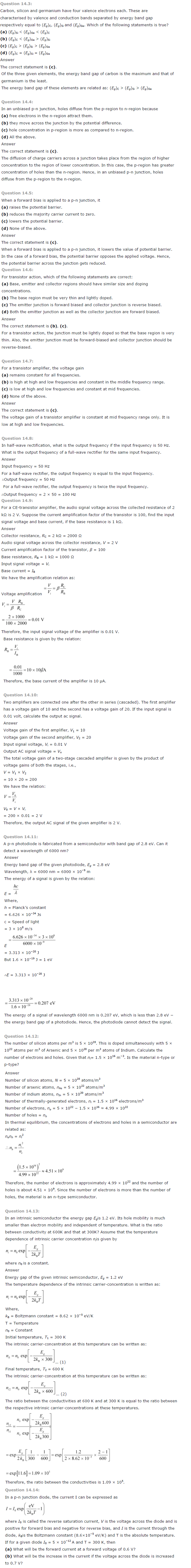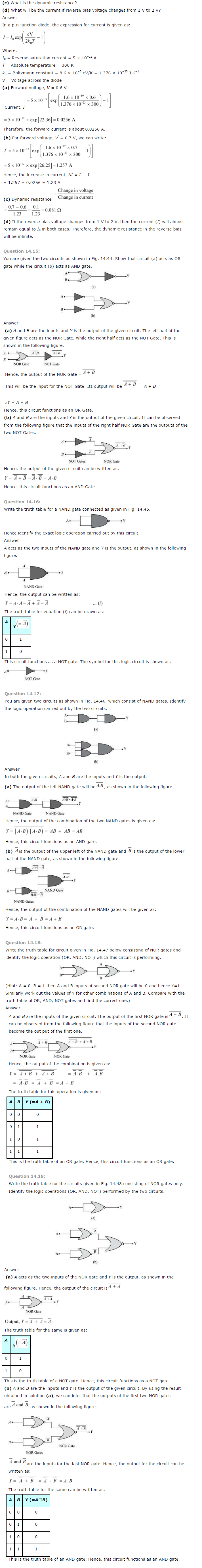### NCERT Solutions for Class 12th Physics Chapter 14: Semiconductor Electronics Materials Devices And Simple Circuits

Question 14.1:
In an n-type silicon, which of the following statement is true:
(a) Electrons are majority carriers and trivalent atoms are the dopants.
(b) Electrons are minority carriers and pentavalent atoms are the dopants.
(c) Holes are minority carriers and pentavalent atoms are the dopants.
(d) Holes are majority carriers and trivalent atoms are the dopants.
The correct statement is (c).
In an n-type silicon, the electrons are the majority carriers, while the holes are the minority carriers. An n-type semiconductor is obtained when pentavalent atoms, such as phosphorus, are doped in silicon atoms.

Question 14.2:
Which of the statements given in Exercise 14.1 is true for p-type semiconductors.
The correct statement is (d).
In a p-type semiconductor, the holes are the majority carriers, while the electrons are the minority carriers. A p-type semiconductor is obtained when trivalent atoms, such as aluminium, are doped in silicon atoms.Powered by: iVerticals Web Technology Pvt Ltd